Home > CC4 > Chapter A > Lesson A.1.8 > ProblemA-82

A-82.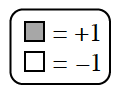Solve the equation represented on each Equation Mats below. Record your work. Check your answer. Homework Help ✎

1.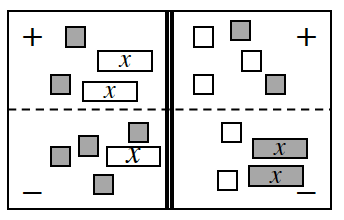A-82a HW eTool (CPM)

2.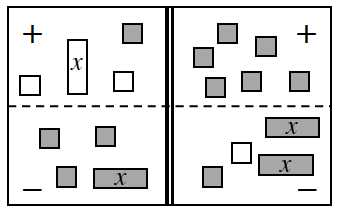A-82b HW eTool (CPM)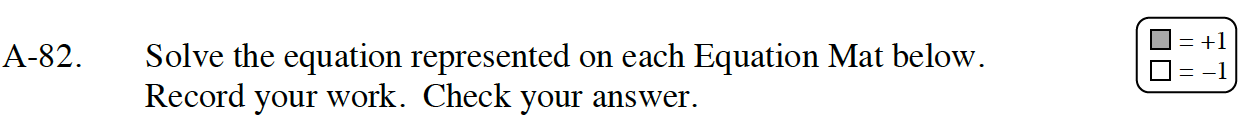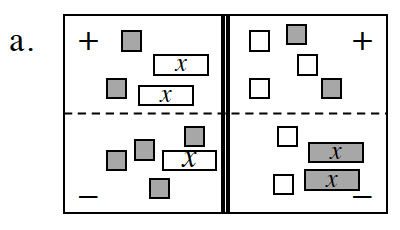Tackle the Equation Mat corner by corner:top left, bottom left, top right, bottom right.
Remember, the problem does not ask you to simplify.

(−2x + 2) − (−x + 4) = ?

Use the Equation Mat below to solve the equation.

Click the link at the right to view the full eTool version: A-82a HW eTool.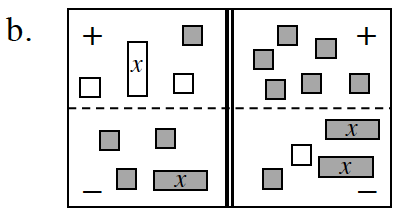Use the Equation Mat below to solve the equation.

Click the link at the right to view the full eTool version: A-82b HW eTool.